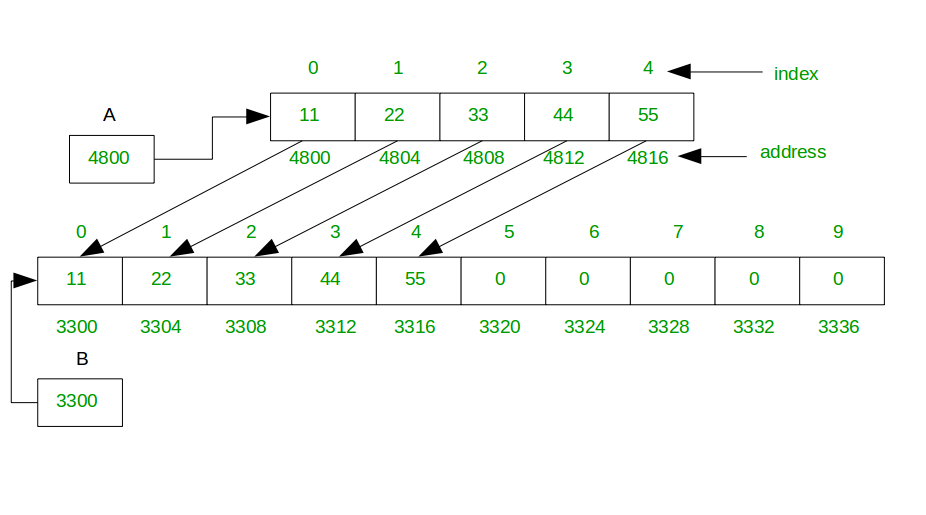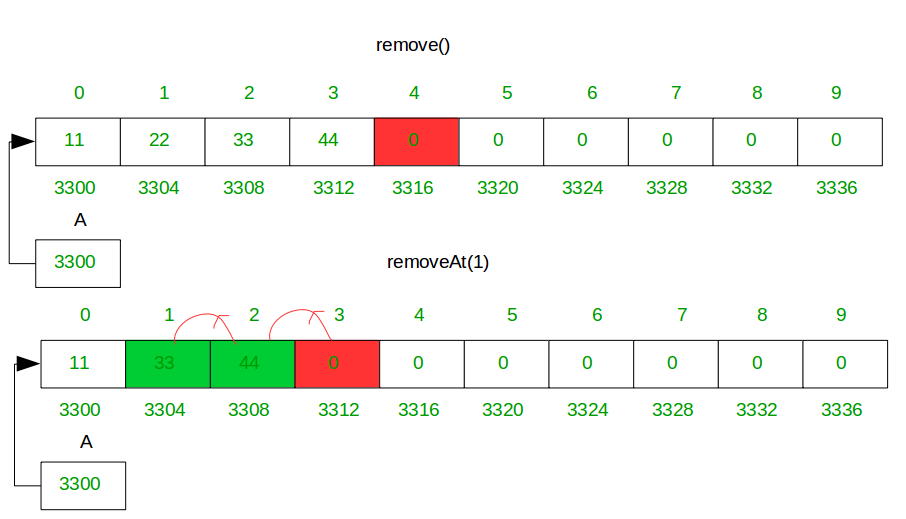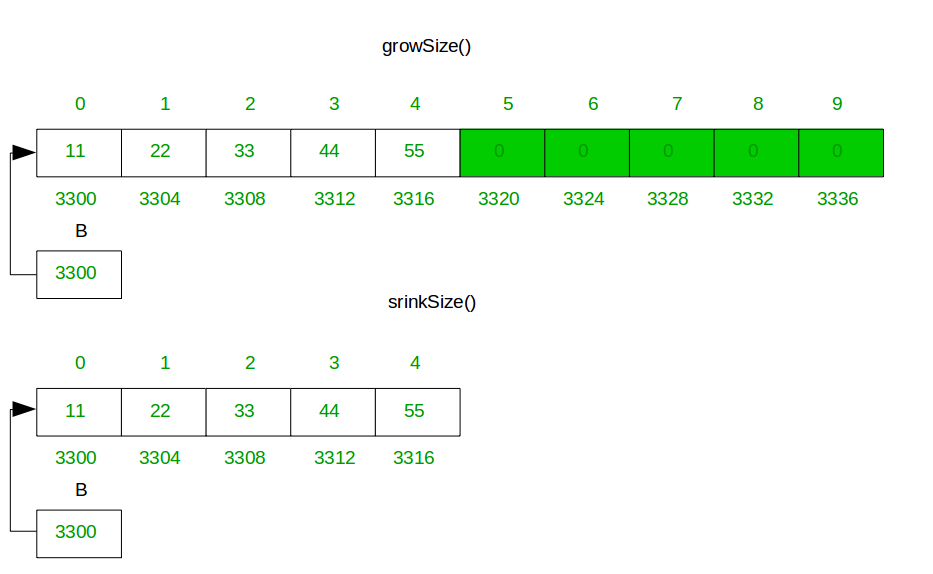Related Articles

# How do Dynamic arrays work?

• Difficulty Level : Medium
• Last Updated : 21 Aug, 2019

A Dynamic array (vector in C++, ArrayList in Java) automatically grows when we try to make an insertion and there is no more space left for the new item. Usually the area doubles in size.

A simple dynamic array can be constructed by allocating an array of fixed-size, typically larger than the number of elements immediately required. The elements of the dynamic array are stored contiguously at the start of the underlying array, and the remaining positions towards the end of the underlying array are reserved, or unused. Elements can be added at the end of a dynamic array in constant time by using the reserved space until this space is completely consumed.

When all space is consumed, and an additional element is to be added, the underlying fixed-sized array needs to be increased in size. Typically resizing is expensive because you have to allocate a bigger array and copy over all of the elements from the array you have overgrow before we can finally append our item.

Approach: When we enter an element in array but array is full then you create a function, this function creates a new array double size or as you wish and copy all element from the previous array to a new array and return this new array. Also, we can reduce the size of the array. and add an element at a given position, remove the element at the end default and at the position also.

### Key Features of Dynamic Array

Add Element: Add element at the end if the array size is not enough then extend the size of the array and add an element at the end of the original array as well as given index. Doing all that copying takes O(n) time, where n is the number of elements in our array. That’s an expensive cost for an append. In a fixed-length array, appends only take O(1) time.

But appends take O(n) time only when we insert into a full array. And that is pretty rare, especially if we double the size of the array every time we run out of space. So in most cases appending is still O(1) time, and sometimes it’s O(n) time.

In dynamic array you can create fixed-size array when required added some more element in array then use this approach:Delete Element: Delete an element from array, default remove() method delete an element from end, simply store zero at last index and you also delete element at specific index by calling removeAt(i) method where i is index. removeAt(i) method shift all right element in the left side from the given index.Resize of Array Size: When the array has null/zero data(exclude add by you) at the right side of the array whose take unrequited memory, the method srinkSize() free extra memory. When all space is consumed, and an additional element is to be added, then the underlying fixed-size array needs to increase size. Typically resizing is expensive because you have to allocate a bigger array and copy over all of the elements from the array you have overgrow before we can finally append our item.Simple code for dynamic array. In below code then the array will become full of size we copy all element to new double size array(variable size array).sample code is below

## Java

 `// Java program deals with all operation of a dynamic array``// add, remove, resize memory of array is the main feature``public` `class` `DynamicArray {`` ` `    ``// create three variable array[] is a array,``    ``// count will deal with no of element add by you and``    ``// size will with size of array[]``    ``private` `int` `array[];``    ``private` `int` `count;``    ``private` `int` `size;``    ``// constructor initialize value to variable`` ` `    ``public` `DynamicArray()``    ``{``        ``array = ``new` `int``[``1``];``        ``count = ``0``;``        ``size = ``1``;``    ``}``    ``// function add an element at the end of array`` ` `    ``public` `void` `add(``int` `data)``    ``{`` ` `        ``// check no of element is equql to size of array``        ``if` `(count == size) {``            ``growSize(); ``// make array size double``        ``} ``// insert element at end of array``        ``array[count] = data;``        ``count++;``    ``}`` ` `    ``// function makes size double of array``    ``public` `void` `growSize()``    ``{`` ` `        ``int` `temp[] = ``null``;``        ``if` `(count == size) {`` ` `            ``// temp is a double size array of array``            ``// and store array elements``            ``temp = ``new` `int``[size * ``2``];``            ``{``                ``for` `(``int` `i = ``0``; i < size; i++) {``                    ``// copy all array value into temp``                    ``temp[i] = array[i];``                ``}``            ``}``        ``}`` ` `        ``// double size array temp initialize``        ``// into variable array again``        ``array = temp;``        ` `        ``// and make size is double also of array``        ``size = size * ``2``;``    ``}`` ` `    ``// function shrink size of array``    ``// which block unnecessary remove them``    ``public` `void` `shrinkSize()``    ``{``        ``int` `temp[] = ``null``;``        ``if` `(count > ``0``) {`` ` `            ``// temp is a count size array``            ``// and store array elements``            ``temp = ``new` `int``[count];``            ``for` `(``int` `i = ``0``; i < count; i++) {`` ` `                ``// copy all array value into temp``                ``temp[i] = array[i];``            ``}`` ` `            ``size = count;`` ` `            ``// count size array temp initialize ``            ``// into variable array again``            ``array = temp;``        ``}``    ``}``    ``// function add an element at given index`` ` `    ``public` `void` `addAt(``int` `index, ``int` `data)``    ``{``        ``// if size is not enough make size double``        ``if` `(count == size) {``            ``growSize();``        ``}`` ` `        ``for` `(``int` `i = count - ``1``; i >= index; i--) {`` ` `            ``// shift all element right ``            ``// from given index``            ``array[i + ``1``] = array[i];``        ``}`` ` `        ``// insert data at given index``        ``array[index] = data;``        ``count++;``    ``}`` ` `    ``// function remove last element or put``    ``// zero at last index``    ``public` `void` `remove()``    ``{``        ``if` `(count > ``0``) {``            ``array[count - ``1``] = ``0``;``            ``count--;``        ``}``    ``}`` ` `    ``// function shift all element of right``    ``// side from given index in left``    ``public` `void` `removeAt(``int` `index)``    ``{``        ``if` `(count > ``0``) {``            ``for` `(``int` `i = index; i < count - ``1``; i++) {`` ` `                ``// shift all element of right ``                ``// side from given index in left``                ``array[i] = array[i + ``1``];``            ``}``            ``array[count - ``1``] = ``0``;``            ``count--;``        ``}``    ``}`` ` `    ``public` `static` `void` `main(String[] args)``    ``{``        ``DynamicArray da = ``new` `DynamicArray();`` ` `        ``// add 9 elements in array``        ``da.add(``1``);``        ``da.add(``2``);``        ``da.add(``3``);``        ``da.add(``4``);``        ``da.add(``5``);``        ``da.add(``6``);``        ``da.add(``7``);``        ``da.add(``8``);``        ``da.add(``9``);`` ` `        ``// print all array elements after add 9 elements``        ``System.out.println(``"Elements of array:"``);``        ``for` `(``int` `i = ``0``; i < da.size; i++) {``            ``System.out.print(da.array[i] + ``" "``);``        ``}`` ` `        ``System.out.println();`` ` `        ``// print size of array and no of element``        ``System.out.println(``"Size of array: "` `+ da.size);``        ``System.out.println(``"No of elements in array: "` `+``                                              ``da.count);`` ` `        ``// shrinkSize of array``        ``da.shrinkSize();`` ` `        ``// print all array elements``        ``System.out.println(``"Elements of array "``+ ``                   ``"after shrinkSize of array:"``);``        ``for` `(``int` `i = ``0``; i < da.size; i++) {``            ``System.out.print(da.array[i] + ``" "``);``        ``}``        ``System.out.println();`` ` `        ``// print size of array and no of element``        ``System.out.println(``"Size of array: "` `+ da.size);``        ``System.out.println(``"No of elements in array: "` `+ ``                                               ``da.count);`` ` `        ``// add an element at index 1``        ``da.addAt(``1``, ``22``);`` ` `        ``// print Elements of array after adding an``        ``// element at index 1``        ``System.out.println(``"Elements of array after"` `+ ``                      ``" add an element at index 1:"``);``        ``for` `(``int` `i = ``0``; i < da.size; i++) {``            ``System.out.print(da.array[i] + ``" "``);``        ``}`` ` `        ``System.out.println();`` ` `        ``// print size of array and no of element``        ``System.out.println(``"Size of array: "` `+ da.size);``        ``System.out.println(``"No of elements in array: "` `+ ``                                               ``da.count);`` ` `        ``// delete last element``        ``da.remove();`` ` `        ``// print Elements of array after delete last ``        ``// element``        ``System.out.println(``"Elements of array after"` `+ ``                              ``" delete last element:"``);``        ``for` `(``int` `i = ``0``; i < da.size; i++) {``            ``System.out.print(da.array[i] + ``" "``);``        ``}`` ` `        ``System.out.println();`` ` `        ``// print size of array and no of element``        ``System.out.println(``"Size of array: "` `+ da.size);``        ``System.out.println(``"No of elements in array: "` `+ ``                                              ``da.count);`` ` `        ``// delete element at index 1``        ``da.removeAt(``1``);`` ` `        ``// print Elements of array after delete``        ``// an element index 1``        ``System.out.println(``"Elements of array after"``+ ``                      ``" delete element at index 1:"``);``        ``for` `(``int` `i = ``0``; i < da.size; i++) {``            ``System.out.print(da.array[i] + ``" "``);``        ``}``        ``System.out.println();`` ` `        ``// print size of array and no of element``        ``System.out.println(``"Size of array: "` `+ da.size);``        ``System.out.println(``"No of elements in array: "` `+``                                               ``da.count);``    ``}``}`

## C#

 `// C# program deals with all operation ``// of dynamic array add, remove, resize ``// memory of array is the main feature``using` `System;`` ` `public` `class` `DynamicArray ``{`` ` `    ``// create three variable array[] is ``    ``// a array, count will deal with no ``    ``// of element add by you and``    ``// size will with size of array[]``    ``private` `int` `[]array;``    ``private` `int` `count;``    ``private` `int` `size;``     ` `    ``// constructor initialize value to variable``    ``public` `DynamicArray()``    ``{``        ``array = ``new` `int``;``        ``count = 0;``        ``size = 1;``    ``}`` ` `    ``// function add an element at the end of array``    ``public` `void` `add(``int` `data)``    ``{`` ` `        ``// check no of element is equql to size of array``        ``if` `(count == size) ``        ``{``            ``growSize(); ``// make array size double``        ``} ``         ` `        ``// insert element at end of array``        ``array[count] = data;``        ``count++;``    ``}`` ` `    ``// function makes size double of array``    ``public` `void` `growSize()``    ``{`` ` `        ``int` `[]temp = ``null``;``        ``if` `(count == size) ``        ``{`` ` `            ``// temp is a double size array of array``            ``// and store array elements``            ``temp = ``new` `int``[size * 2];``            ``{``                ``for` `(``int` `i = 0; i < size; i++) ``                ``{``                    ``// copy all array value into temp``                    ``temp[i] = array[i];``                ``}``            ``}``        ``}`` ` `        ``// double size array temp initialize``        ``// into variable array again``        ``array = temp;``         ` `        ``// and make size is double also of array``        ``size = size * 2;``    ``}`` ` `    ``// function shrink size of array``    ``// which block unnecessary remove them``    ``public` `void` `shrinkSize()``    ``{``        ``int` `[]temp = ``null``;``        ``if` `(count > 0) ``        ``{`` ` `            ``// temp is a count size array``            ``// and store array elements``            ``temp = ``new` `int``[count];``            ``for` `(``int` `i = 0; i < count; i++) ``            ``{`` ` `                ``// copy all array value into temp``                ``temp[i] = array[i];``            ``}`` ` `            ``size = count;`` ` `            ``// count size array temp initialize ``            ``// into variable array again``            ``array = temp;``        ``}``    ``}``     ` `    ``// function add an element at given index``    ``public` `void` `addAt(``int` `index, ``int` `data)``    ``{``        ``// if size is not enough make size double``        ``if` `(count == size)``        ``{``            ``growSize();``        ``}`` ` `        ``for` `(``int` `i = count - 1; i >= index; i--)``        ``{`` ` `            ``// shift all element right ``            ``// from given index``            ``array[i + 1] = array[i];``        ``}`` ` `        ``// insert data at given index``        ``array[index] = data;``        ``count++;``    ``}`` ` `    ``// function remove last element or put``    ``// zero at last index``    ``public` `void` `remove()``    ``{``        ``if` `(count > 0) ``        ``{``            ``array[count - 1] = 0;``            ``count--;``        ``}``    ``}`` ` `    ``// function shift all element of right``    ``// side from given index in left``    ``public` `void` `removeAt(``int` `index)``    ``{``        ``if` `(count > 0)``        ``{``            ``for` `(``int` `i = index; i < count - 1; i++) ``            ``{`` ` `                ``// shift all element of right ``                ``// side from given index in left``                ``array[i] = array[i + 1];``            ``}``            ``array[count - 1] = 0;``            ``count--;``        ``}``    ``}`` ` `    ``// Driver code``    ``public` `static` `void` `Main()``    ``{``        ``DynamicArray da = ``new` `DynamicArray();`` ` `        ``// add 9 elements in array``        ``da.add(1);``        ``da.add(2);``        ``da.add(3);``        ``da.add(4);``        ``da.add(5);``        ``da.add(6);``        ``da.add(7);``        ``da.add(8);``        ``da.add(9);`` ` `        ``// print all array elements after add 9 elements``        ``Console.WriteLine(``"Elements of array:"``);``        ``for` `(``int` `i = 0; i < da.size; i++) ``        ``{``            ``Console.Write(da.array[i] + ``" "``);``        ``}`` ` `        ``Console.WriteLine();`` ` `        ``// print size of array and no of element``        ``Console.WriteLine(``"Size of array: "` `+ da.size);``        ``Console.WriteLine(``"No of elements in array: "` `+``                                            ``da.count);`` ` `        ``// shrinkSize of array``        ``da.shrinkSize();`` ` `        ``// print all array elements``        ``Console.WriteLine(``"Elements of array "``+ ``                ``"after shrinkSize of array:"``);``        ``for` `(``int` `i = 0; i < da.size; i++) ``        ``{``            ``Console.Write(da.array[i] + ``" "``);``        ``}``        ``Console.WriteLine();`` ` `        ``// print size of array and no of element``        ``Console.WriteLine(``"Size of array: "` `+ da.size);``        ``Console.WriteLine(``"No of elements in array: "` `+ ``                                            ``da.count);`` ` `        ``// add an element at index 1``        ``da.addAt(1, 22);`` ` `        ``// print Elements of array after adding an``        ``// element at index 1``        ``Console.WriteLine(``"Elements of array after"` `+ ``                    ``" add an element at index 1:"``);``        ``for` `(``int` `i = 0; i < da.size; i++) ``        ``{``            ``Console.Write(da.array[i] + ``" "``);``        ``}`` ` `        ``Console.WriteLine();`` ` `        ``// print size of array and no of element``        ``Console.WriteLine(``"Size of array: "` `+ da.size);``        ``Console.WriteLine(``"No of elements in array: "` `+ ``                                            ``da.count);`` ` `        ``// delete last element``        ``da.remove();`` ` `        ``// print Elements of array after delete last ``        ``// element``        ``Console.WriteLine(``"Elements of array after"` `+ ``                            ``" delete last element:"``);``        ``for` `(``int` `i = 0; i < da.size; i++) ``        ``{``            ``Console.Write(da.array[i] + ``" "``);``        ``}`` ` `        ``Console.WriteLine();`` ` `        ``// print size of array and no of element``        ``Console.WriteLine(``"Size of array: "` `+ da.size);``        ``Console.WriteLine(``"No of elements in array: "` `+ ``                                            ``da.count);`` ` `        ``// delete element at index 1``        ``da.removeAt(1);`` ` `        ``// print Elements of array after delete``        ``// an element index 1``        ``Console.WriteLine(``"Elements of array after"``+ ``                    ``" delete element at index 1:"``);``        ``for` `(``int` `i = 0; i < da.size; i++) ``        ``{``            ``Console.Write(da.array[i] + ``" "``);``        ``}``        ``Console.WriteLine();`` ` `        ``// print size of array and no of element``        ``Console.WriteLine(``"Size of array: "` `+ da.size);``        ``Console.WriteLine(``"No of elements in array: "` `+``                                            ``da.count);``    ``}``}`` ` `/* This code contributed by PrinciRaj1992 */`
Output:
```Elements of array:
1 2 3 4 5 6 7 8 9 0 0 0 0 0 0 0
Size of array: 16
No of elements in array: 9
Elements of array after shrinkSize of array:
1 2 3 4 5 6 7 8 9
Size of array: 9
No of elements in array: 9
Elements of array after add an element at index 1:
1 22 2 3 4 5 6 7 8 9 0 0 0 0 0 0 0 0
Size of array: 18
No of elements in array: 10
Elements of array after delete last element:
1 22 2 3 4 5 6 7 8 0 0 0 0 0 0 0 0 0
Size of array: 18
No of elements in array: 9
Elements of array after delete element at index 1:
1 2 3 4 5 6 7 8 0 0 0 0 0 0 0 0 0 0
Size of array: 18
No of elements in array: 8
```

Attention reader! Don’t stop learning now. Get hold of all the important DSA concepts with the DSA Self Paced Course at a student-friendly price and become industry ready.  To complete your preparation from learning a language to DS Algo and many more,  please refer Complete Interview Preparation Course.

In case you wish to attend live classes with experts, please refer DSA Live Classes for Working Professionals and Competitive Programming Live for Students.

My Personal Notes arrow_drop_up